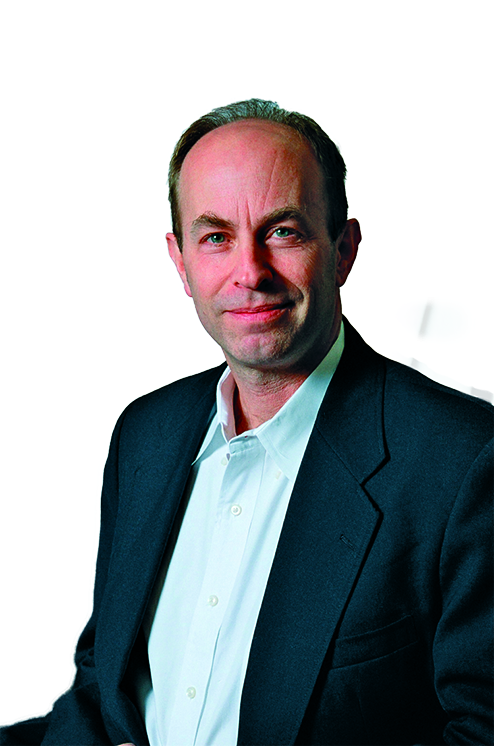# Algorithm is faster than FFT for all sparse signals

Feb. 9, 2012
Researchers at the Massachusetts Institute of technology (Cambridge, MA) have developed two algorithms that are faster than the fast Fourier transform (FFT) for all sparse signals.

Being able to compute the Fourier transform of an input signal is crucial in photonics—for example, in determining the spatial frequencies in an image. The standard method of computing a discrete Fourier transform (DFT) is by using the fast Fourier transform (FFT) algorithm. However, algorithms faster than the FFT would be desirable. Researchers at the Massachusetts Institute of technology (Cambridge, MA) have developed two algorithms that are faster than the FFT for all sparse signals. (A sparse signal is one in which some of its Fourier coefficients are near enough to zero that they can be ignored.) While other algorithms have previously been developed to improve on the FFT for sparse signals, none of them have improved on the FFT’s runtime for the whole range of sparse signals.

For a signal with k nonzero Fourier coefficients, and a length n of the input signal that is a power of 2, the researchers show two new DFT algorithms. The first is an O(k log n)-time algorithm for the exactly k-sparse case (where k is small). (O means “on the order of.”) The second is an O(k log n log(n/k))-time algorithm for the general case. In contrast, the FFT computes the DFT in O(n log n) time. Contact Haitham Hassanieh at [email protected].### John Wallace | Senior Technical Editor (1998-2022)

John Wallace was with Laser Focus World for nearly 25 years, retiring in late June 2022. He obtained a bachelor's degree in mechanical engineering and physics at Rutgers University and a master's in optical engineering at the University of Rochester. Before becoming an editor, John worked as an engineer at RCA, Exxon, Eastman Kodak, and GCA Corporation.

### What AI demands mean for data centers

Nov. 28, 2023
The 2023 Photonics-Enabled Cloud Computing Summit assembled by Optica took an aggressive approach to calling out the limitations of today’s current technologies.

### Chroma Customer Spotlight - Dr. David Warshaw, About his Lab

Nov. 27, 2023
David Warshaw, Professor and Chair of Molecular Physiology and Biophysics at the University of Vermont (UVM), walks us through his lab. Learn about his lab’s work with the protein...